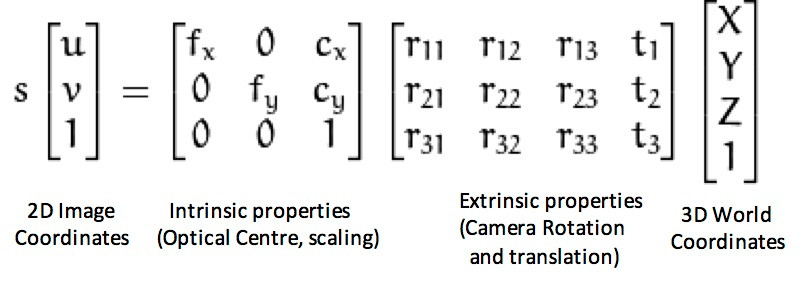Camera Calibration

# Calibration Matrix §

This matrix only represents the Camera Intrinsics.

### Most Common Notation §

Kajanan told me that the most common form is

K = \begin{equation} \begin{bmatrix} f_x & s&c_x \\ 0& f_y& c_y\\ 0&0 & 1 \end{bmatrix} \end{equation}

• is the shear factor
• ​ and ​ represent the focal length of the camera in terms of pixels for the and axes
• ​ and ​ are the coordinates of the Principal Point

### Cyrill Notation §

Through Cyrill Stachniss course, I see it through (see Camera Calibration)

so we end up with this calibration matrix

• is the distance between the camera origin and the image plane
• scale difference between and
• Sheer compensation (for digital cameras, we typically have )

So we essentially have this additional scale difference through the Cyrill Stachniss notation, but the scale difference is generally , so we have